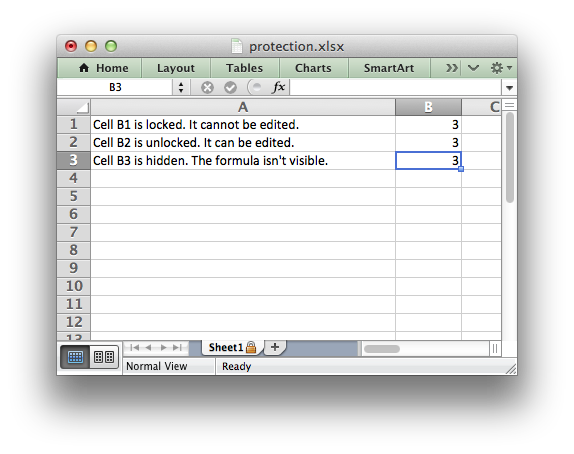# Example: Enabling Cell protection in Worksheets#

This program is an example cell locking and formula hiding in an Excel worksheet using the `protect()` worksheet method and the Format `set_locked()` property.

Note, that Excel’s behavior is that all cells are locked once you set the default protection. Therefore you need to explicitly unlock cells rather than explicitly lock them.```########################################################################
#
# Example of cell locking and formula hiding in an Excel worksheet
# using Python and the XlsxWriter module.
#
# Copyright 2013-2023, John McNamara, jmcnamara@cpan.org
#
import xlsxwriter

workbook = xlsxwriter.Workbook("protection.xlsx")

# Create some cell formats with protection properties.

# Format the columns to make the text more visible.
worksheet.set_column("A:A", 40)

# Turn worksheet protection on.
worksheet.protect()

# Write a locked, unlocked and hidden cell.
worksheet.write("A1", "Cell B1 is locked. It cannot be edited.")
worksheet.write("A2", "Cell B2 is unlocked. It can be edited.")
worksheet.write("A3", "Cell B3 is hidden. The formula isn't visible.")

worksheet.write_formula("B1", "=1+2")  # Locked by default.
worksheet.write_formula("B2", "=1+2", unlocked)
worksheet.write_formula("B3", "=1+2", hidden)

workbook.close()
```